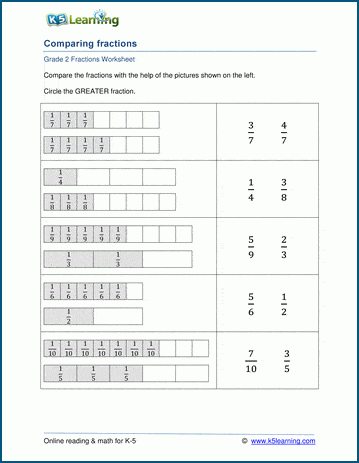# Comparing fractions with block diagrams

## Block diagram worksheets: which fraction is greater?

These worksheets ask students to compare fractions with different numerators and denominators by visualizing the fractions as blocks of the same size split into different numbers of equal parts.## More fractions worksheets

Explore all of our fractions worksheets, from dividing shapes into "equal parts" to multiplying and dividing improper fractions and mixed numbers.

## What is K5?

K5 Learning offers reading and math worksheets, workbooks and an online reading and math program for kids in kindergarten to grade 5.  We help your children build good study habits and excel in school.